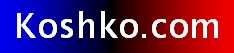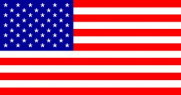The personal web site of Rick Koshko (married name Rick Wiegmann Koshko). To email me, click here for the email form. I currently use these social networks: LinkedIn, Twitter, Facebook. My compendium of links to useful, interesting, and cool web sites.To contact me Click here for the email form.## Day-of-the-Week finder

This fairly easy to memorize day-of-the-week finding formula was printed in The Teacher Brothers' Modern-Day Almanac 1984 (Running Press, Philadelphia). The acknowledgements credit it to Jean-Claude Bailiff's Superpuzzles (Prentice-Hall, 1982). The almanac's version of the formula lacks a century correction and was presented as correct only for the 20th Century (1900 to 1999, not the true Century). It works for Gregorian dates only, but it shouldn't be hard to modify for Julian Calendar dates. I also wrote a JavaScript version to do the math for you.

 Determine the month code. Not 1 through 12. But from the following: Jan = 0 Feb = 3 Mar = 3 Apr = 6 May = 1 Jun = 4 Jul = 6 Aug = 2 Sep = 5 Oct = 0 Nov = 3 Dec = 5 Add the date (1 to 31). Subtract 1 if the date falls within January or February of a leap year. Add the last two digits of the year. Take the last two digits of the year, divide by four and drop the fraction. Add the result to the running tally. Add the appropriate century correction: 1600's, 2000's, 2400's, 2800's = 6 1700's, 2100's, 2500's, 2900's = 4 1800's, 2200's, 2600's, 3000's = 2 1900's, 2300's, 2700's, 3100's = 0 Take the grand total from your running tally and divide by seven.

The remainder indicates the day of the week.

0=Sun 1=Mon 2=Tue 3=Wed 4=Thu 5=Fri 6=Sat

Example: What day was April 18, 1886?

 April's month code is 6 6 The 18th 18 Not January or February of a leap year - 0 Last two digits of 1886 86 86 ÷ 4 = 21 (and a fraction) 21 1800's century correction = 2 2 Grand total 133 133 ÷ 7 19 and no remainder Remainder of zero means... Sunday